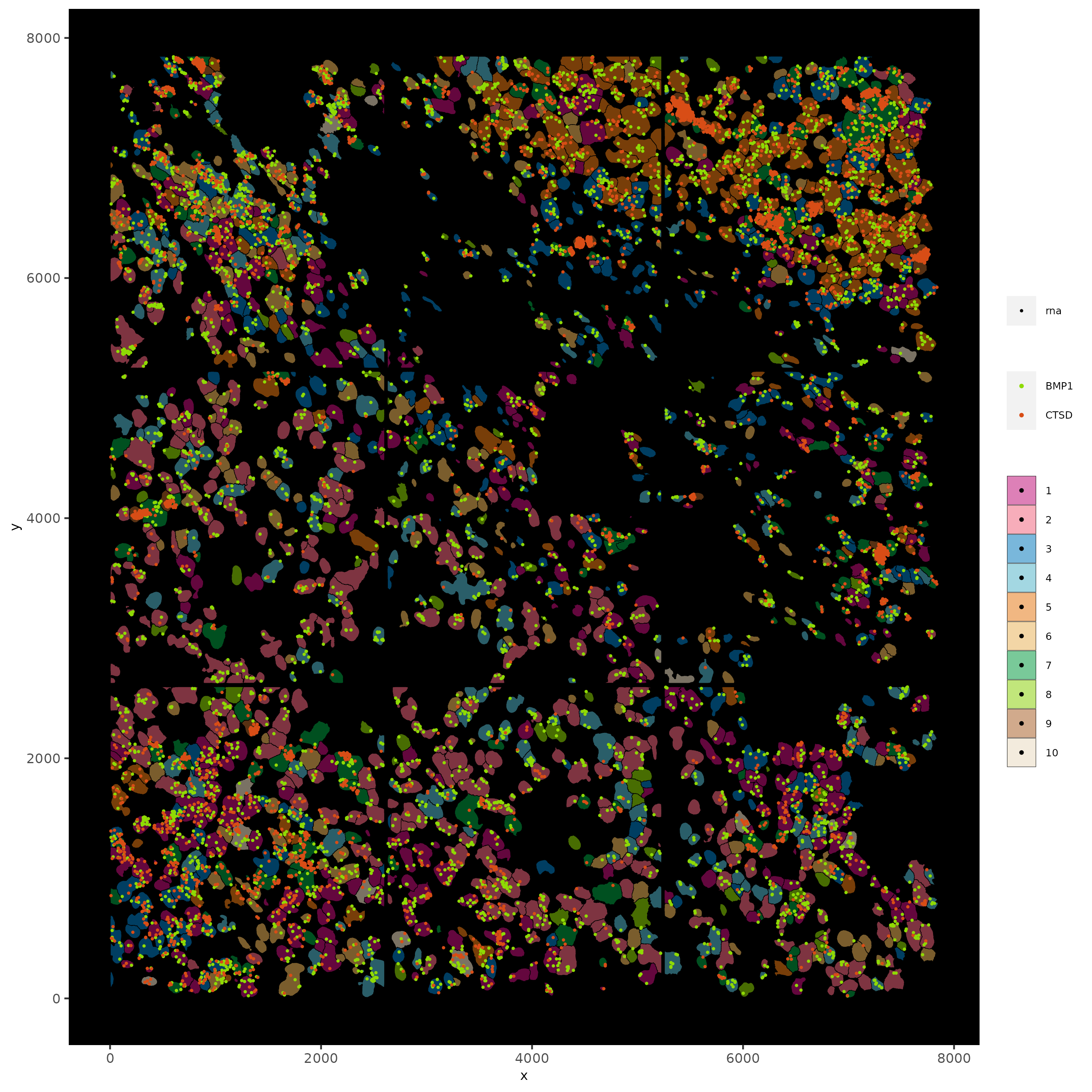# Resolve Bioscience Breast Cancer Subcellular#

```# Ensure Giotto Suite is installed.
if(!"Giotto" %in% installed.packages()) {
devtools::install_github("drieslab/Giotto@suite")
}

# Ensure GiottoData, a small, helper module for tutorials, is installed.
if(!"GiottoData" %in% installed.packages()) {
devtools::install_github("drieslab/GiottoData")
}
library(Giotto)
# Ensure the Python environment for Giotto has been installed.
genv_exists = checkGiottoEnvironment()
if(!genv_exists){
# The following command need only be run once to install the Giotto environment.
installGiottoEnvironment()
}
```

## Start Giotto#

```library(Giotto)
library(GiottoData)

# 1. set working directory
my_working_dir = '/path/to/directory'

# Optional: Specify a path to a Python executable within a conda or miniconda
# environment. If set to NULL (default), the Python executable within the previously
# installed Giotto environment will be used.
my_python_path = NULL # alternatively, "/local/python/path/python" if desired.
```

## Input Files#

```## provide path to resolve bioscience folder
data_path = '/path/to/Resolve_bioscience_cancer'

# 1. original image as png
original_DAPI_image = paste0(data_path, '/', 'sample2_DAPI.jpg')

# 2. input cell segmentation as mask file
# can also be provided as a 3-column polygon file
# to be used as image background AND to store segmentations as polygons
# can be obtained through Fiji / QuPath / Ilastik / Cellpose / ...

# 3. input features coordinates
colnames(tx_coord) = c('x', 'y', 'z', 'gene_id')
tx_coord = tx_coord[,.(x, y, gene_id)]
```

## Part 1: Create Subcellular Giotto Object#

```testobj = createGiottoObjectSubcellular(gpoints = list('rna' = tx_coord),
instructions = instrs,
verbose = FALSE,
cores = 32)
```

## Part 2: Create Spatial Locations#

```# centroids are now used to provide the spatial locations (centroid of each cell)
# needed for certain downstream spatial analyses
poly_info = 'cell')
```

## Part 3: Add Image Information#

```# create Giotto images
DAPI_image = createGiottoImage(gobject = testobj,
name = 'DAPI',
image_transformations = 'flip_x_axis',
mg_object = original_DAPI_image)

segm_image = createGiottoImage(gobject = testobj,
name = 'segmentation',
image_transformations = 'flip_x_axis',

# add images to Giotto object
images = list(DAPI_image, segm_image))

# provides an overview of available images
showGiottoImageNames(testobj)
```

## Part 4: Visualize Original Images#

```# visualize overlay of calculated cell centroid with original image and segmentation mask file
# by setting show_plot to FALSE and save_plot to TRUE you can save quite some time when creating plots
# with big images it sometimes takes quite long for R/Rstudio to render them
spatPlot2D(gobject = testobj, image_name = 'DAPI', point_size = 1.5)
```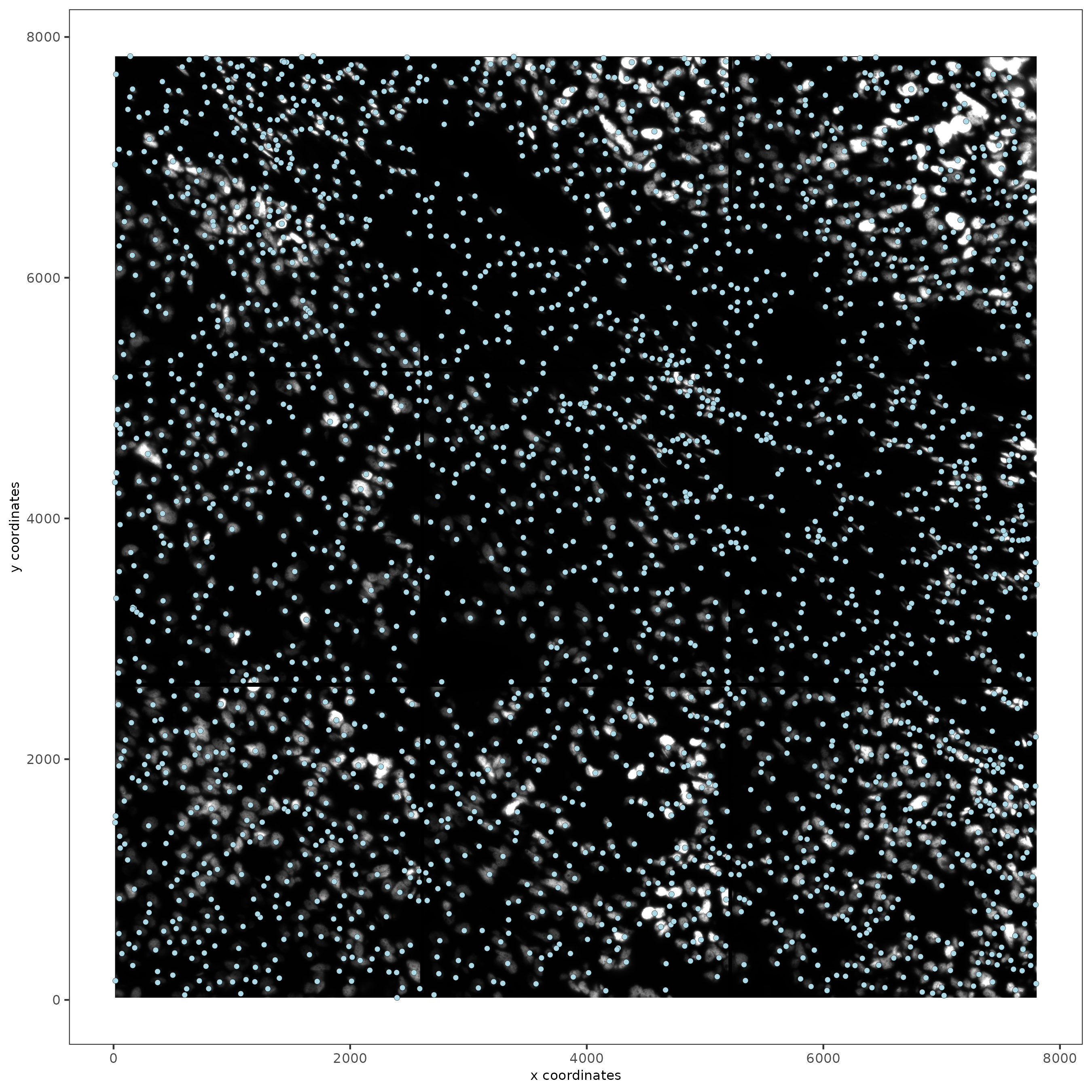```spatPlot2D(gobject = testobj, image_name = 'segmentation', point_size = 1.5)
```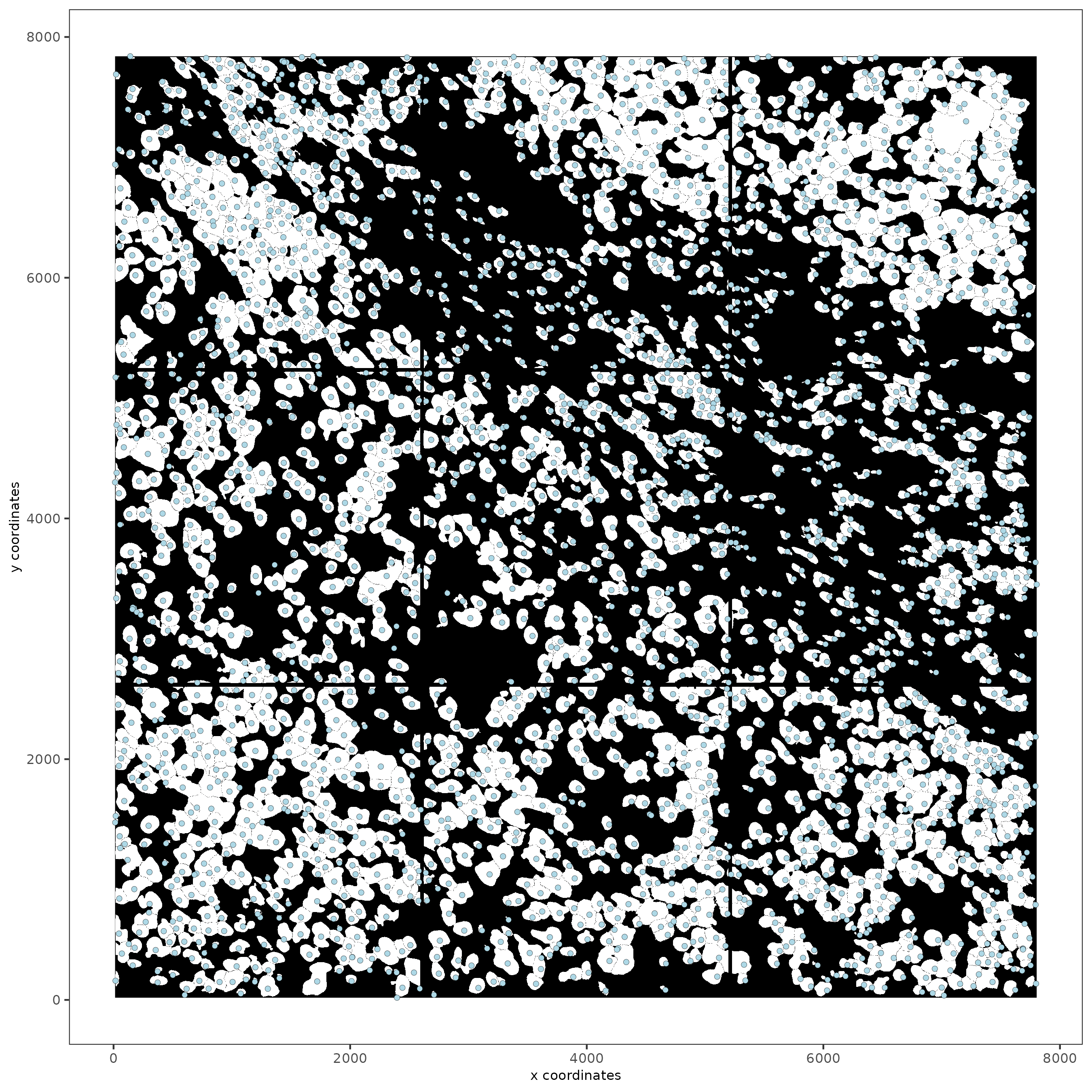## Part 5: Calculate Cell Shape Overlap#

```tictoc::tic()
testobj = calculateOverlap(testobj,
method = 'parallel',
x_step = 1000,
y_step = 1000,
poly_info = 'cell',
feat_info = 'rna')
tictoc::toc()
#convert overlap to matrix
testobj = overlapToMatrix(testobj,
poly_info = 'cell',
feat_info = 'rna',
name = 'raw')
```

## Part 6: Filter Data#

```# features can be filtered individually
# cells will be filtered across features

# first filter on rna
subc_test <- filterGiotto(gobject = testobj,
expression_threshold = 1,
feat_det_in_min_cells = 20,
min_det_feats_per_cell = 5)

spatPlot2D(gobject = subc_test,
image_name = 'segmentation', show_image = TRUE,
point_size = 1.5)
```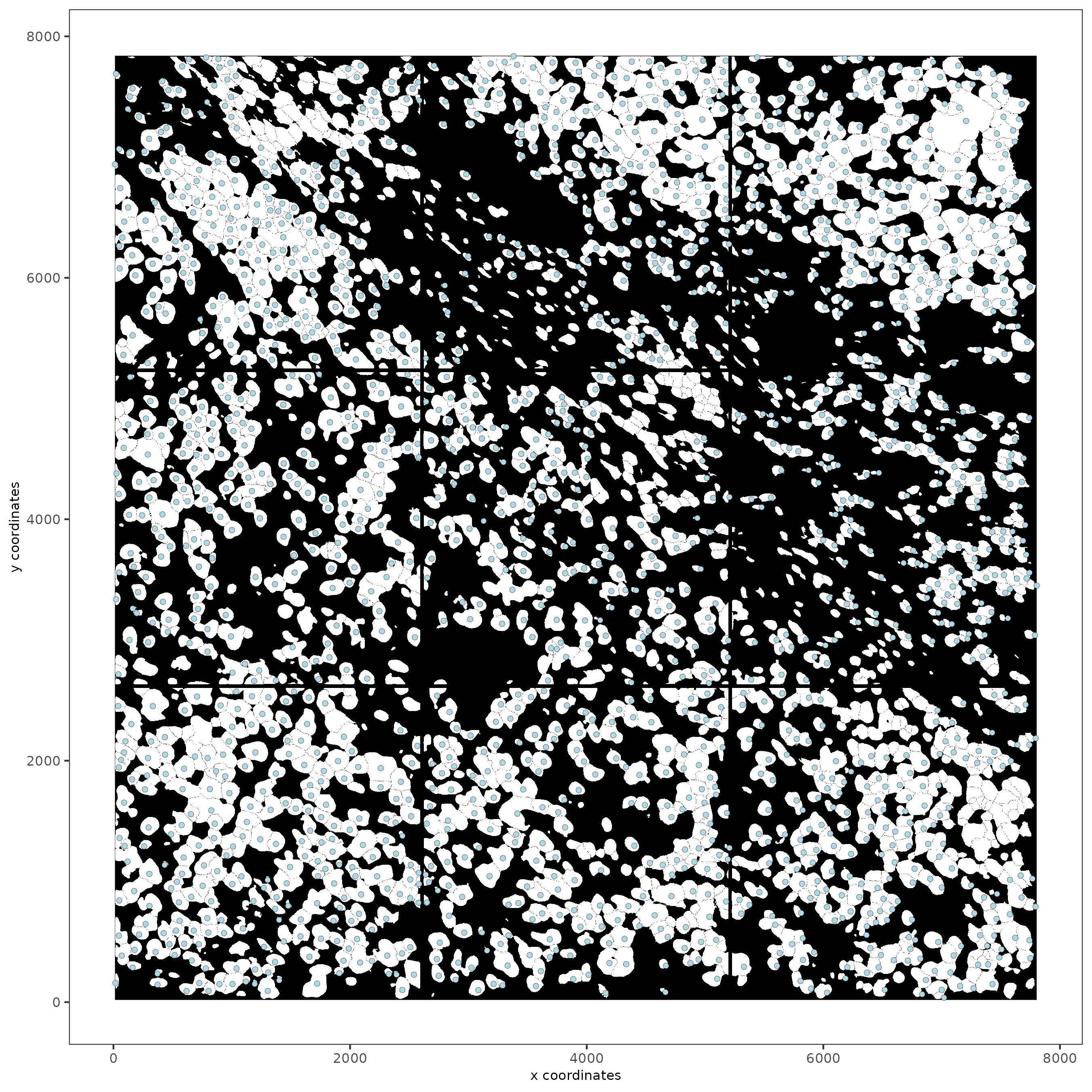```# rna data, default.
# other feature modalities can be processed and filtered in an anologous manner
subc_test <- normalizeGiotto(gobject = subc_test, scalefactor = 6000, verbose = T)
expression_values = c('normalized'),
covariate_columns = c('nr_feats', 'total_expr'))

subc_test <- normalizeGiotto(gobject = subc_test, norm_methods = 'pearson_resid', update_slot = 'pearson')
showGiottoExpression(subc_test)
```

## Part 8: Dimension Reduction#

```# Find highly valuable Features

# typical way of calculating HVF
subc_test <- calculateHVF(gobject = subc_test, HVFname= 'hvg_orig')
```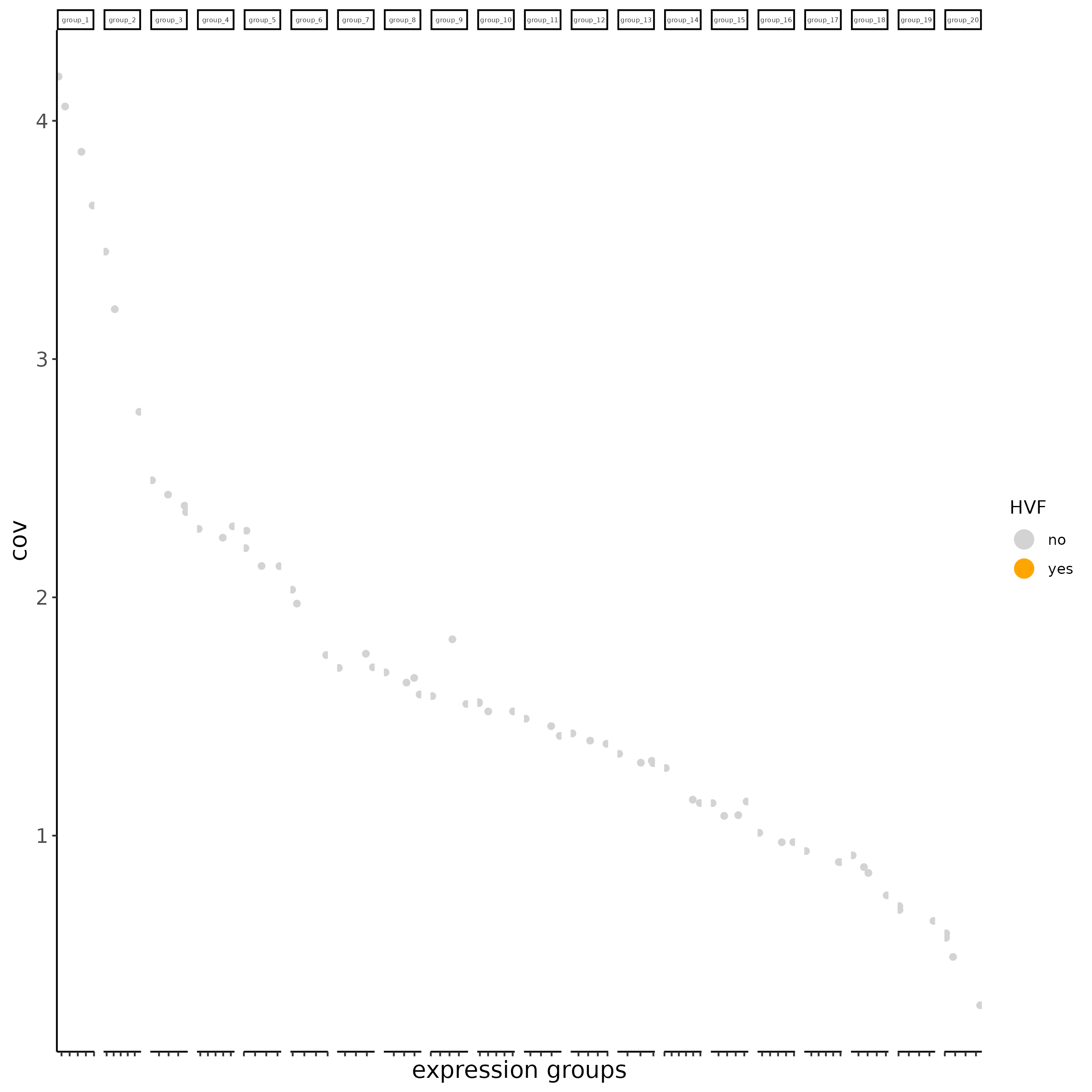```# new method based on variance of pearson residuals for each gene
subc_test <- calculateHVF(gobject = subc_test,
method = 'var_p_resid', expression_values = 'pearson',
show_plot = T)
```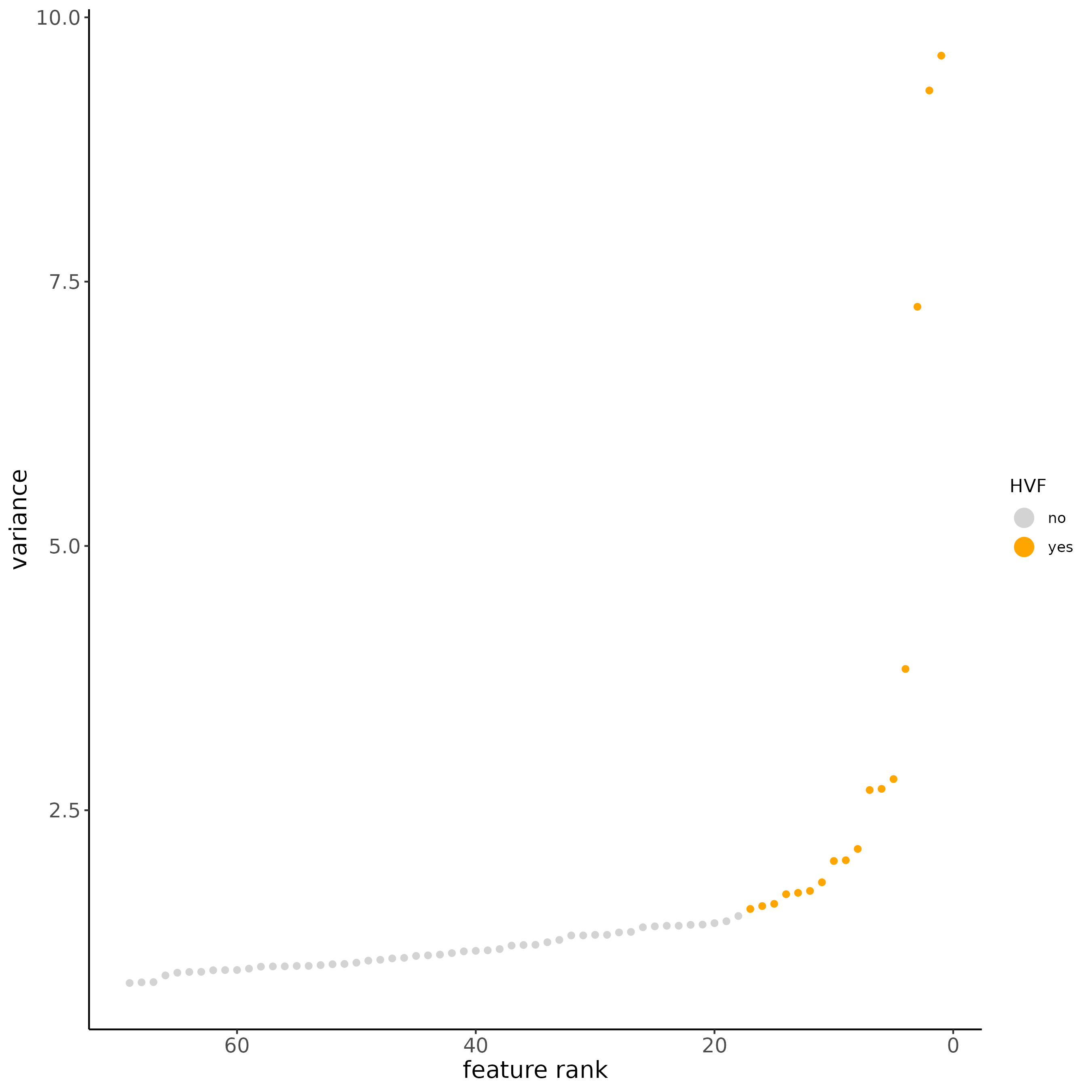```#run PCA
subc_test <- runPCA(gobject = subc_test,
expression_values = 'pearson',
scale_unit = F, center = F)

screePlot(subc_test, ncp = 20)
```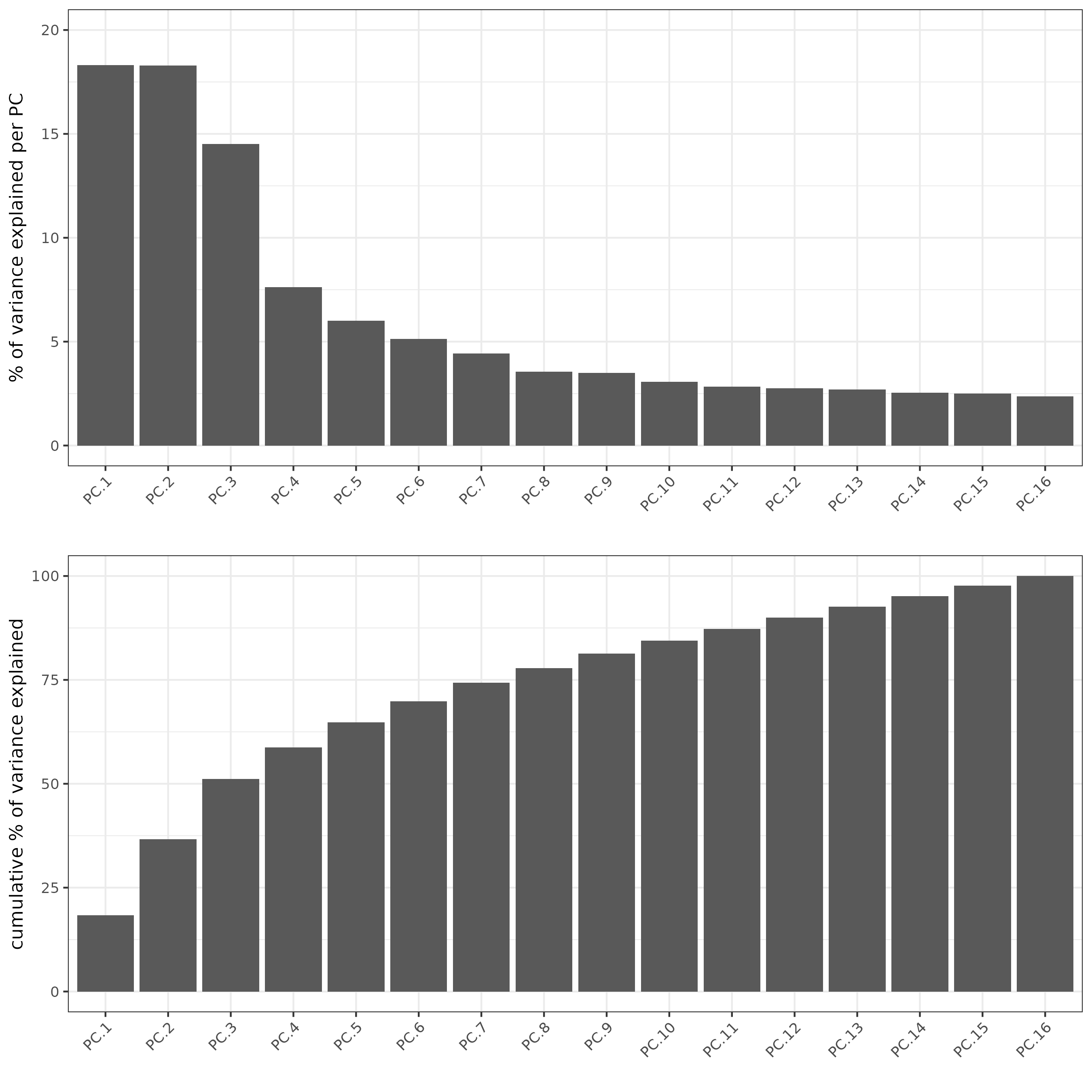```plotPCA(subc_test,
dim1_to_use = 1,
dim2_to_use = 2)
```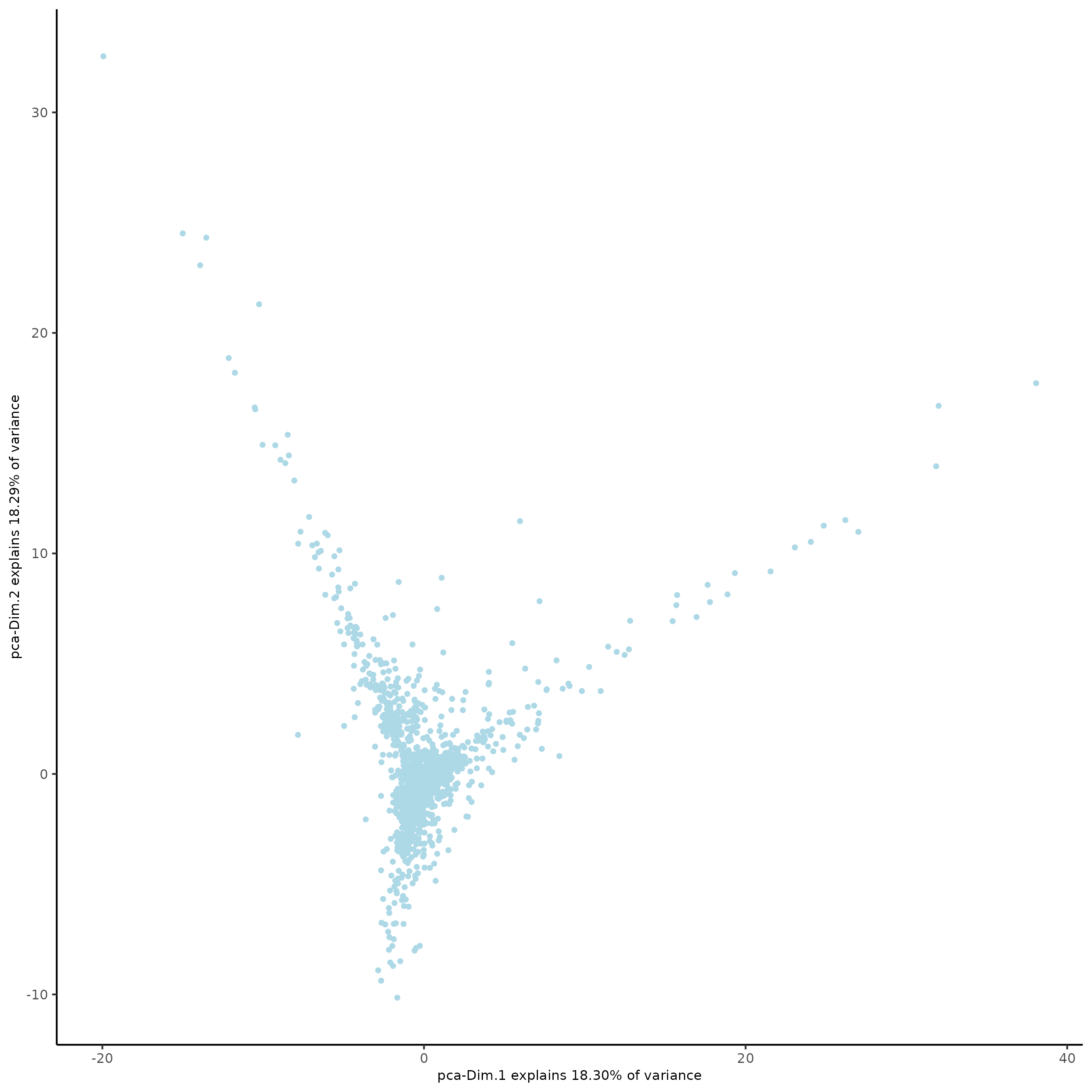```# run UMAP
subc_test <- runUMAP(subc_test, dimensions_to_use = 1:5, n_threads = 2)
plotUMAP(gobject = subc_test)
```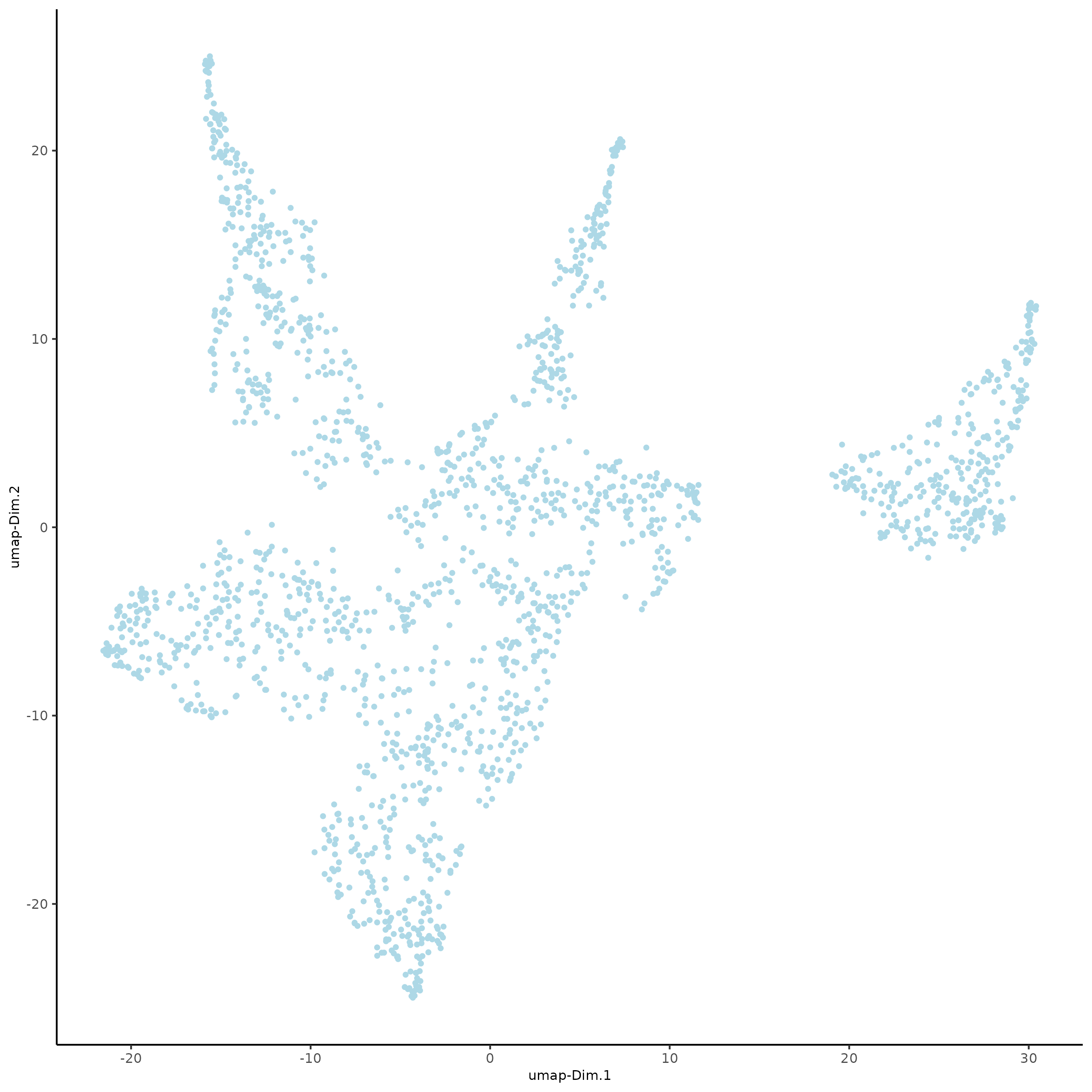## Part 9: Cluster#

```subc_test <- createNearestNetwork(gobject = subc_test, dimensions_to_use = 1:5, k = 5)
subc_test <- doLeidenCluster(gobject = subc_test, resolution = 0.05, n_iterations = 1000, name = 'leiden_0.05')

# Create color palettes, or proceed with Giotto defaults
devtools::install_github("alyssafrazee/RSkittleBrewer")
colorcode = lacroix_palette(type = "paired")
featcolor = lacroix_palette("KeyLime", type = "discrete")

# visualize UMAP cluster results
plotUMAP(gobject = subc_test, cell_color = 'leiden_clus',
show_NN_network = T, point_size = 2.5, cell_color_code = colorcode)
```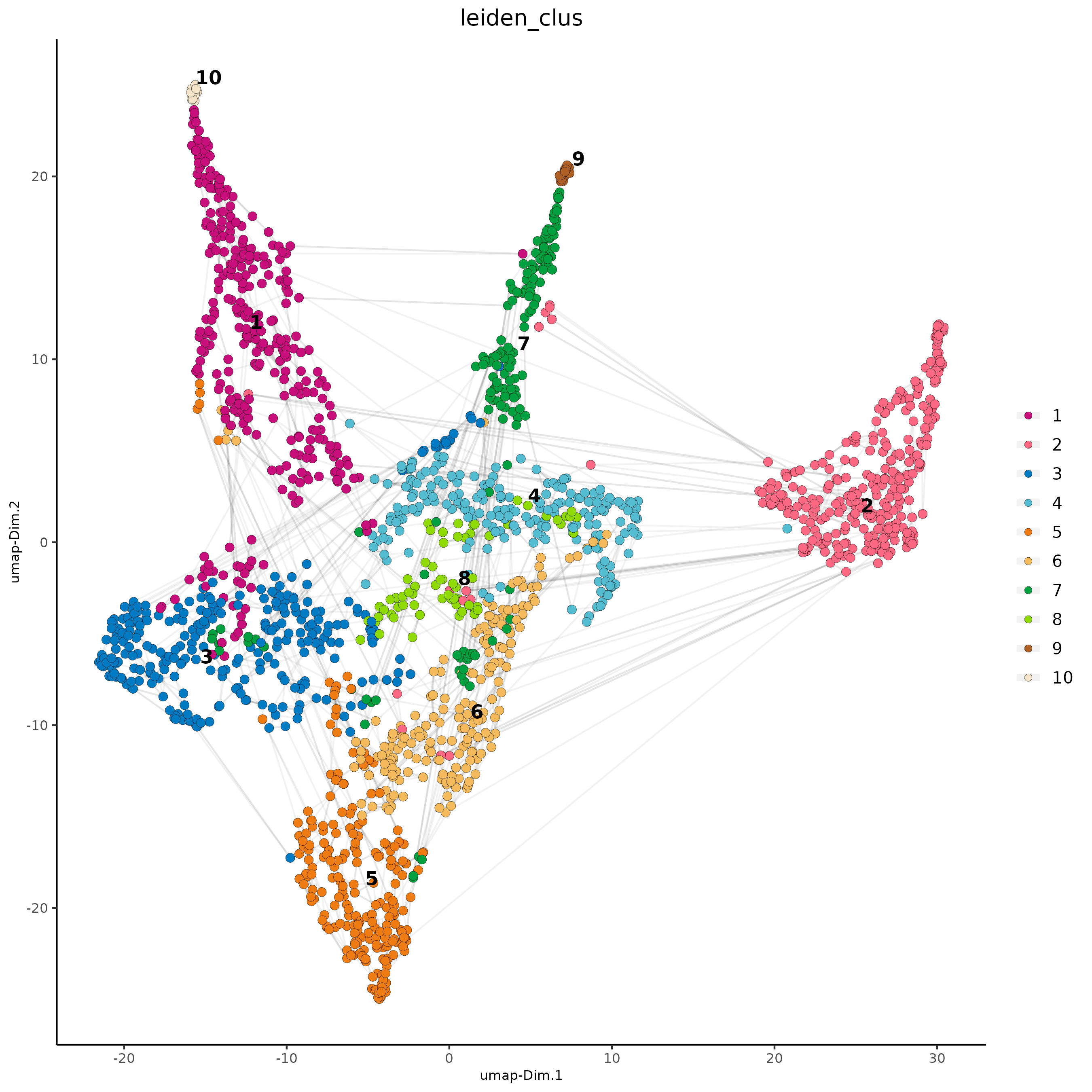```# visualize UMAP and spatial results
spatDimPlot2D(gobject = subc_test,
show_image = T, image_name = 'segmentation',
cell_color = 'leiden_clus',
spat_point_size = 2, cell_color_code = colorcode)
```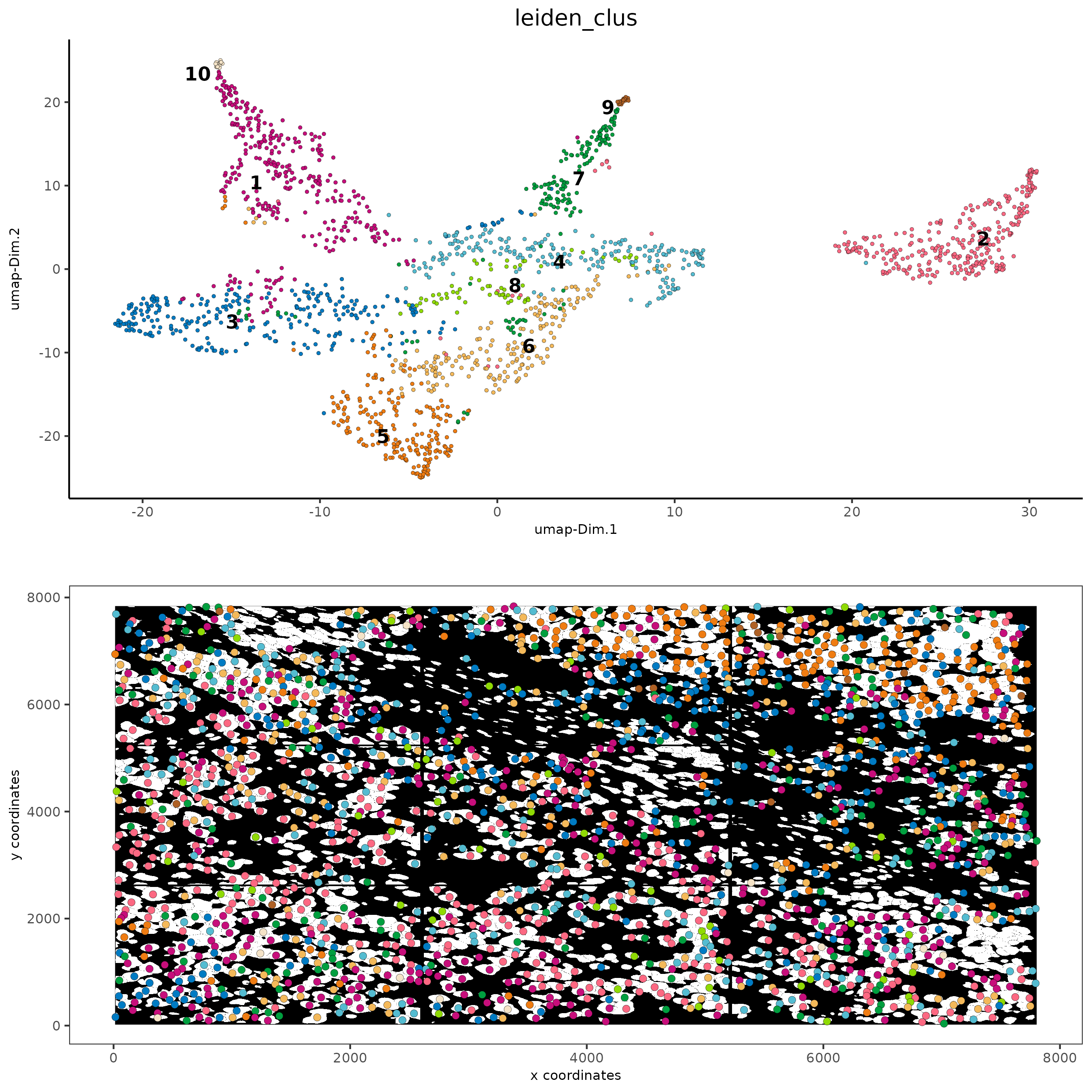```# Plot a cluster heatmap
showClusterHeatmap(gobject = subc_test, cluster_column = 'leiden_clus',
save_param = list(save_format = 'pdf',base_height = 6, base_width = 8, units = 'cm'))
```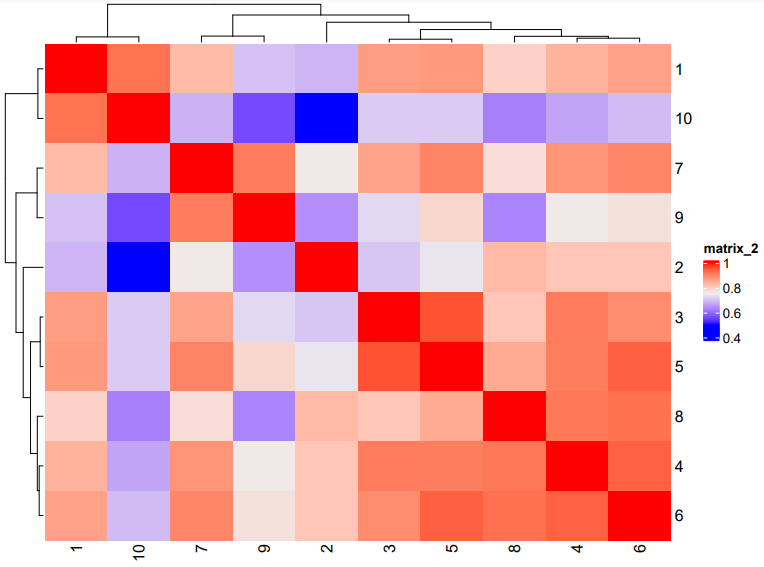```# See cluster relationships in a dendogram
showClusterDendrogram(subc_test, h = 0.5, rotate = T, cluster_column = 'leiden_clus')
```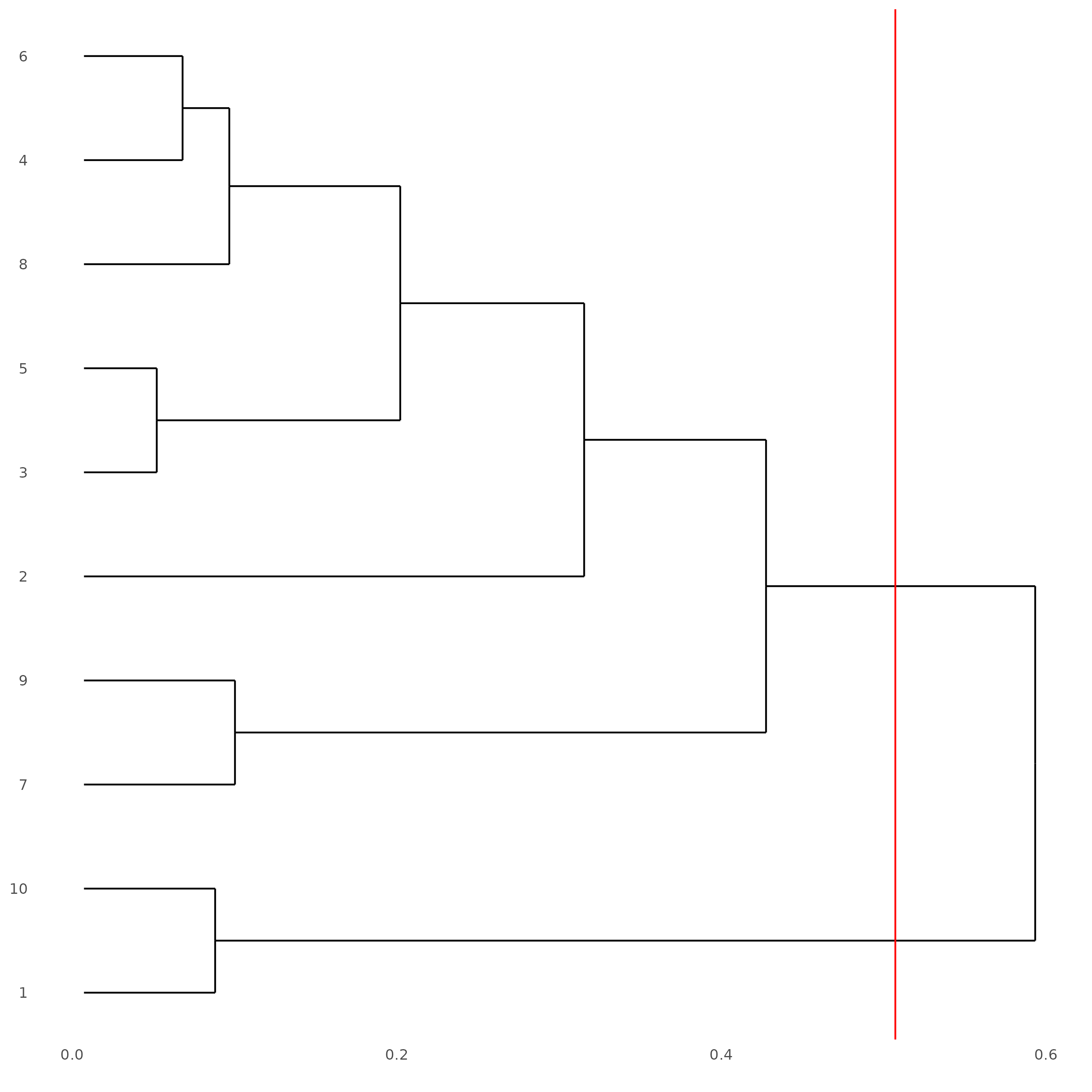## Part 10: Create a Spatial Network#

```subc_test = createSpatialNetwork(gobject = subc_test,
spat_loc_name = 'cell',
minimum_k = 3,
maximum_distance_delaunay = 100)

spatPlot2D(gobject = subc_test,
image_name = 'segmentation', show_image = TRUE,
point_size = 1.5, show_network = TRUE)
```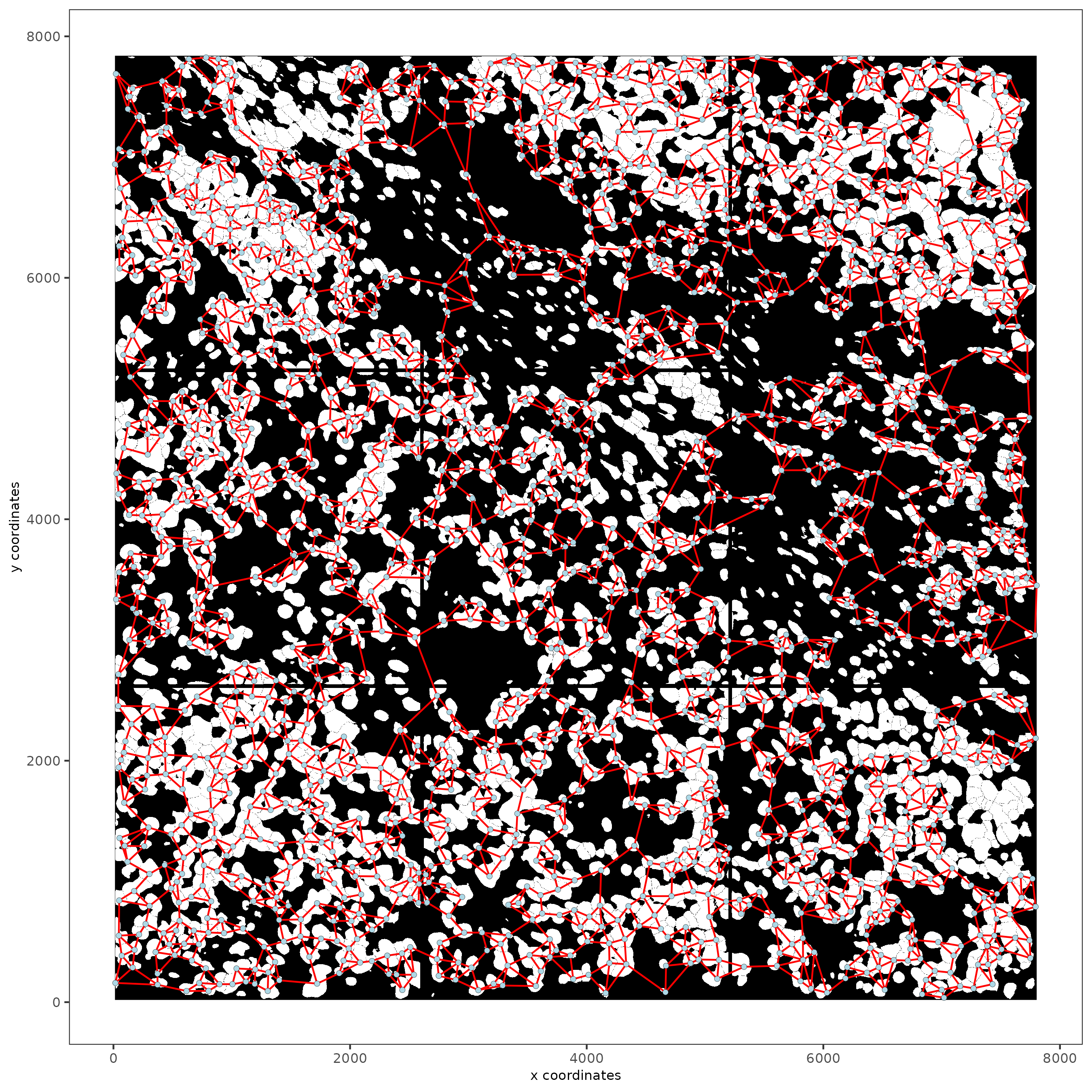## Part 11: Visualize SubCellular Data#

```# Visualize clustered cells
spatInSituPlotPoints(subc_test,
show_polygon = TRUE,
polygon_feat_type = 'cell',
polygon_color = 'white',
polygon_line_size = 0.1,
polygon_fill = 'leiden_clus',
polygon_fill_as_factor = T ,
polygon_fill_code = colorcode)
```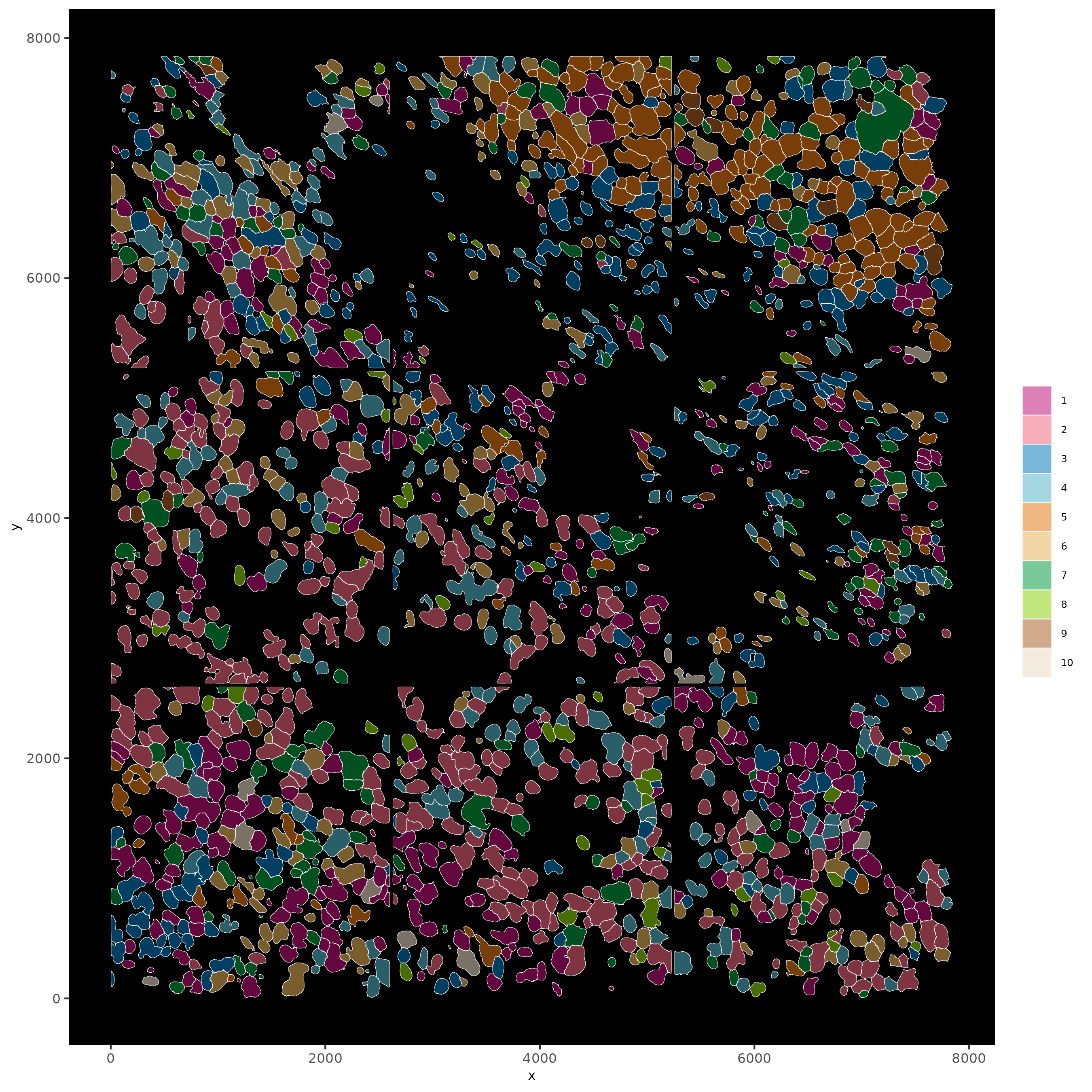```# individual plotting of transcripts and polygon information

# all cells
spatInSituPlotPoints(testobj,
feats = list('rna' = c("MMP2", "VEGFA", "IGF1R", 'CDH2', 'MKI67')),
point_size = 0.2,
show_polygon = TRUE,
polygon_feat_type = 'cell',
polygon_color = 'white',
polygon_line_size = 0.1)
```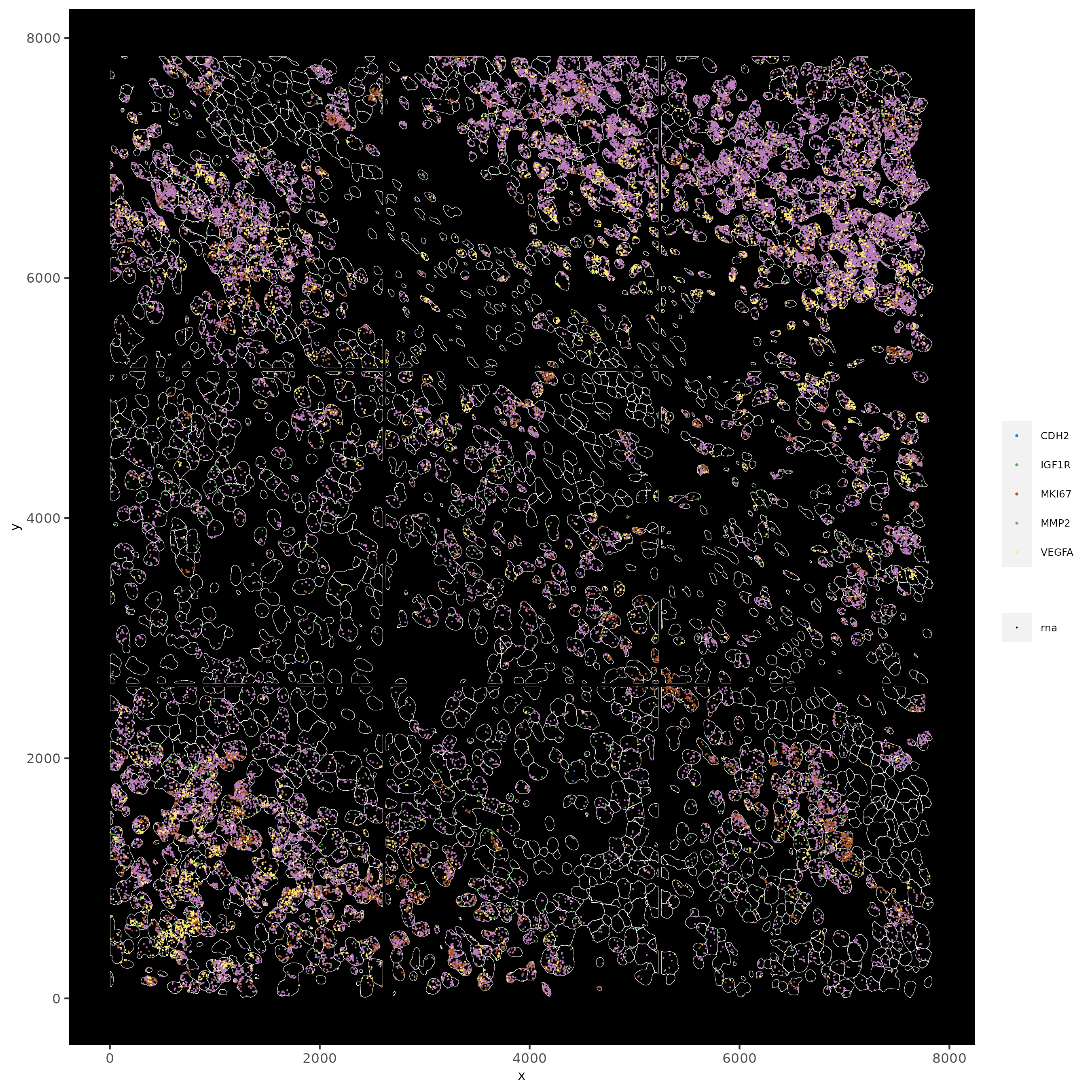```# filtered cells
spatInSituPlotPoints(subc_test,
feats = list('rna' = c("MMP2", "VEGFA", "IGF1R", 'CDH2', 'MKI67')),
point_size = 0.2,
show_polygon = TRUE,
polygon_feat_type = 'cell',
polygon_color = 'white',
polygon_line_size = 0.1)
```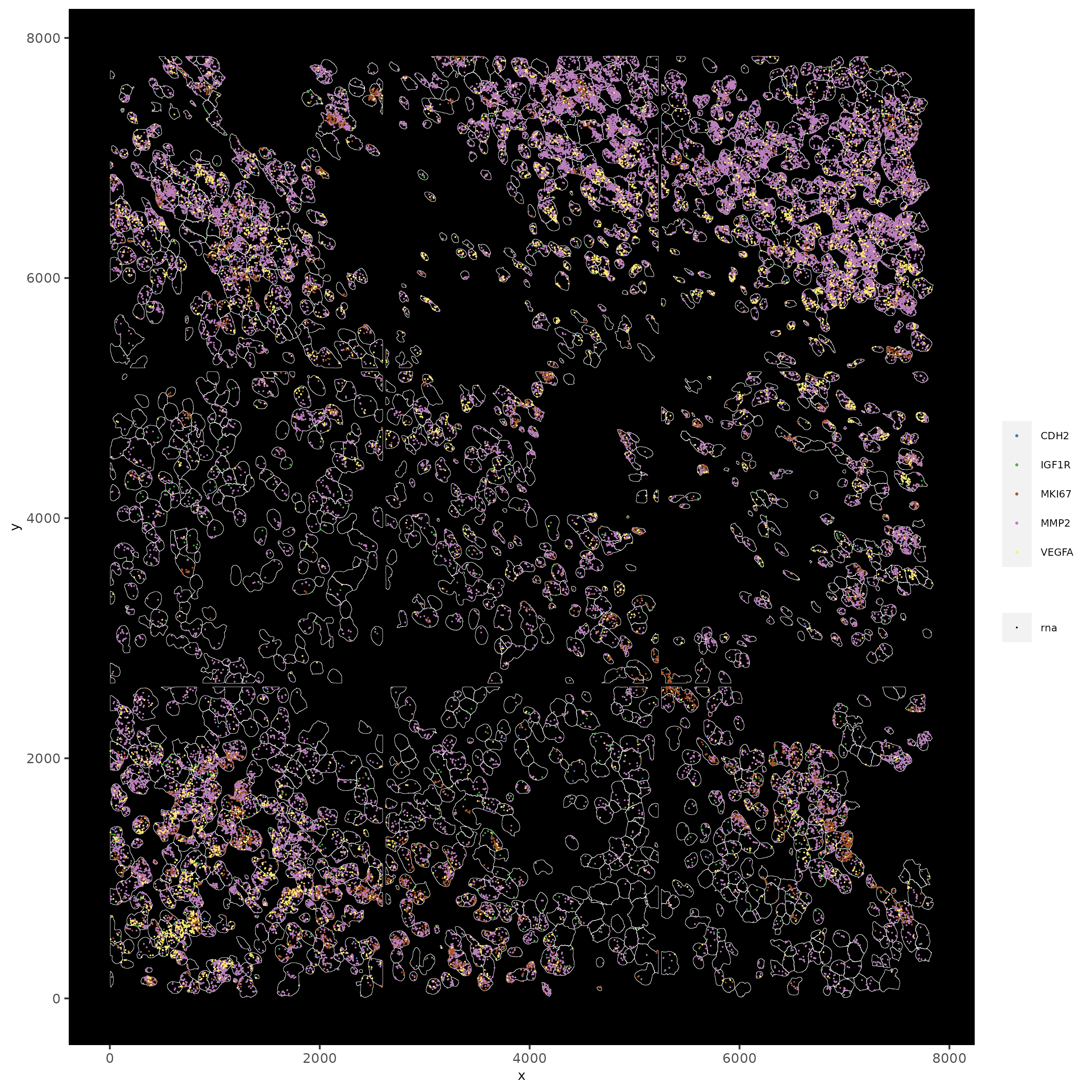```# faster plotting method if you have many points
spatInSituPlotPoints(subc_test,
plot_method = 'scattermore',
feats = list('rna' = c("MMP2", "VEGFA", "IGF1R", 'CDH2', 'MKI67')),
point_size = 0.2,
show_polygon = TRUE,
polygon_feat_type = 'cell',
polygon_color = 'white',
polygon_line_size = 0.1)
```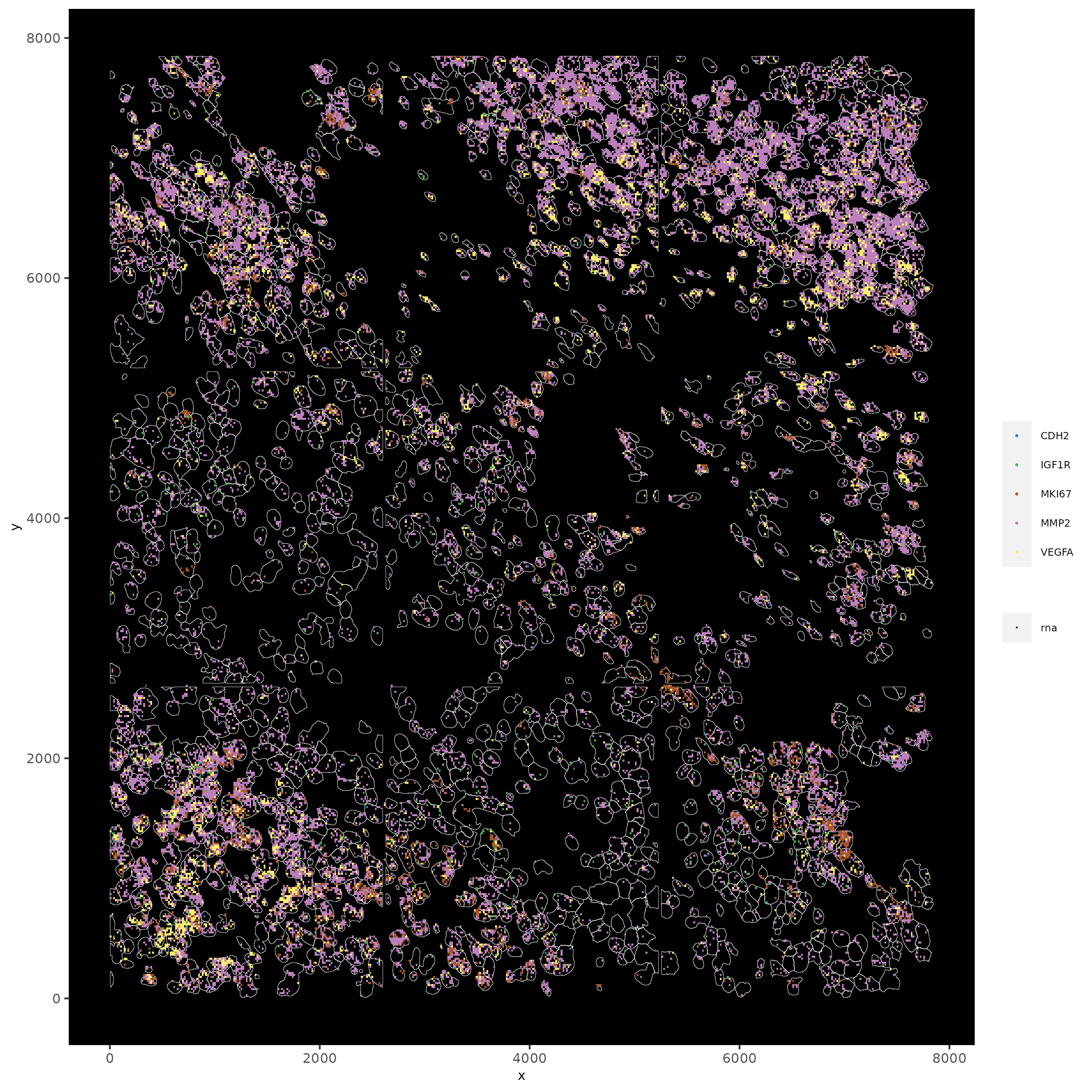### 11.1 Subset by Location#

```# can be used to focus on specific spatial structures
# to zoom in on niche environments

subloc = subsetGiottoLocs(subc_test,
x_min = 0, x_max = 2000,
y_min = 0, y_max = 2000,
poly_info = 'cell')

# show subset of genes
spatInSituPlotPoints(subloc,
feats = list('rna' = c("MMP2", "VEGFA", "IGF1R", 'CDH2', 'MKI67')),
point_size = 0.6,
show_polygon = TRUE,
polygon_feat_type = 'cell',
polygon_color = 'white',
polygon_line_size = 0.1)
```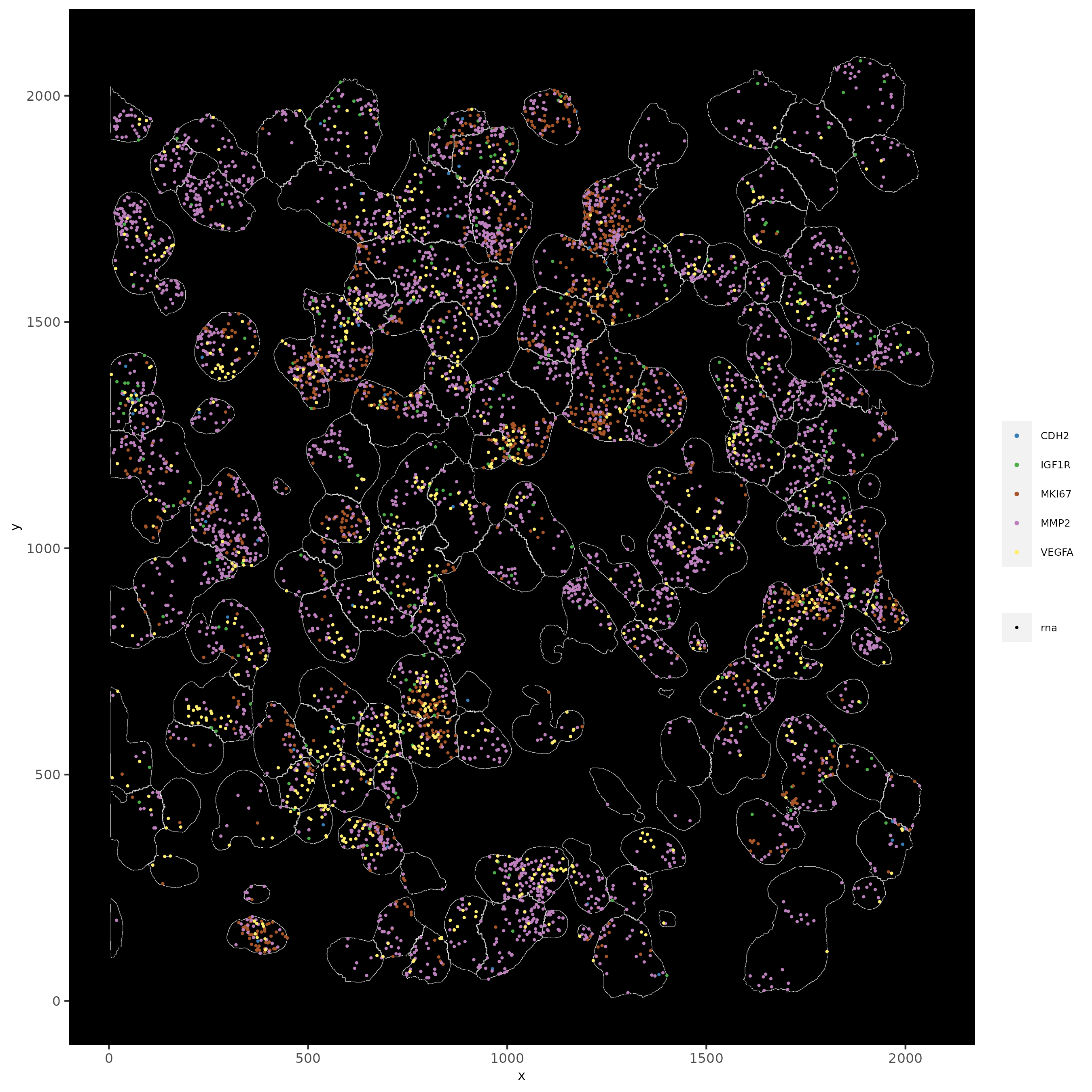```# show subset of genes and color cells according to clusters
spatInSituPlotPoints(subloc,
feats = list('rna' = c("MMP2", "VEGFA", "IGF1R", 'CDH2', 'MKI67')),
point_size = 0.6,
show_polygon = TRUE,
polygon_feat_type = 'cell',
polygon_color = 'white',
polygon_line_size = 0.1,
polygon_fill = 'leiden_clus',
polygon_fill_as_factor = T,
polygon_fill_code = colorcode,
feats_color_code = featcolor)
```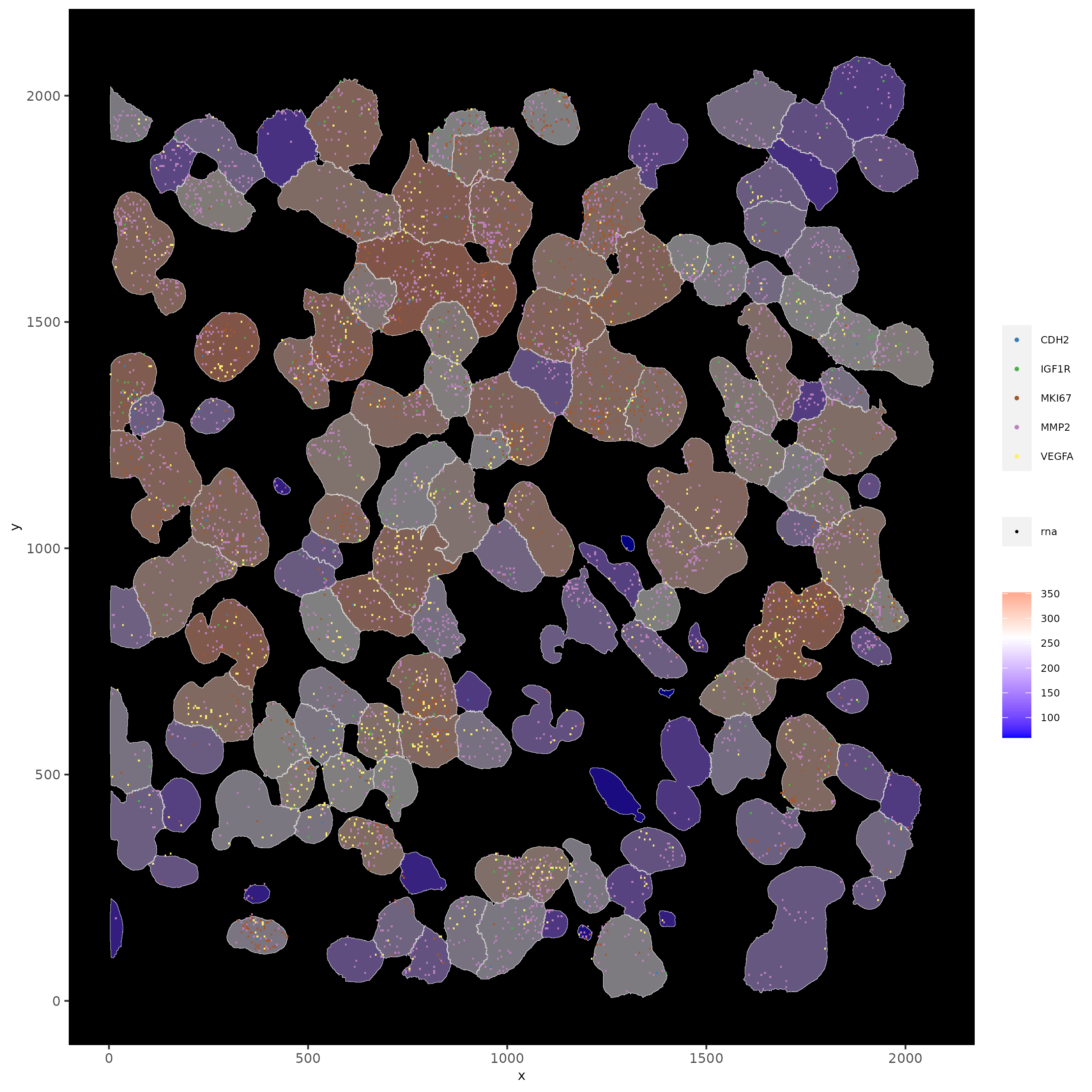```# show subset of genes and color cells according to total expression
# use a faster and more efficient point plotting method = scattermore
spatInSituPlotPoints(subloc,
plot_method = 'scattermore',
feats = list('rna' = c("MMP2", "VEGFA", "IGF1R", 'CDH2', 'MKI67')),
point_size = 0.6,
show_polygon = TRUE,
polygon_feat_type = 'cell',
polygon_color = 'white',
polygon_line_size = 0.1,
polygon_fill = 'total_expr',
polygon_fill_as_factor = F)
```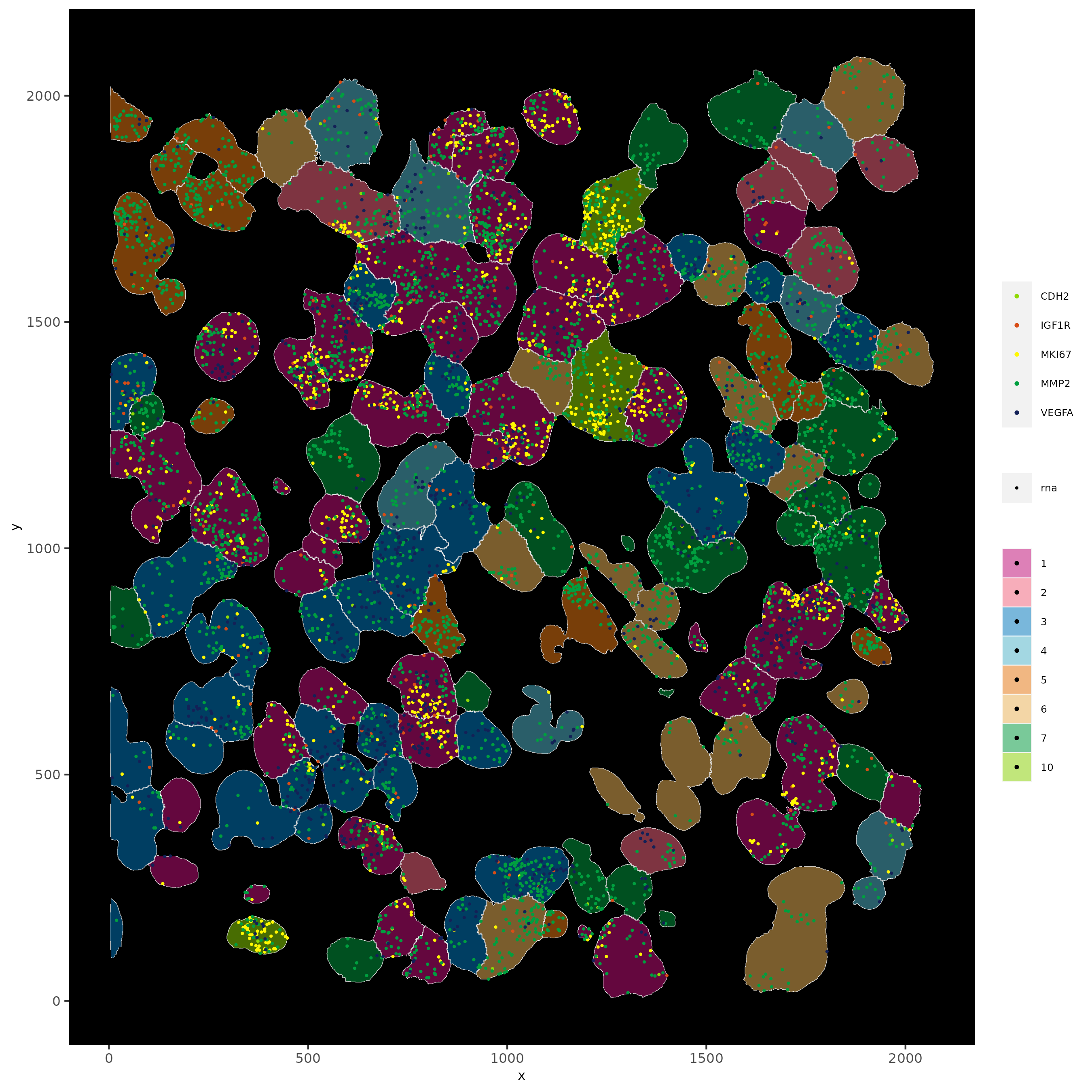```# show cells and color them according to total expression
spatInSituPlotPoints(subloc,
show_polygon = TRUE,
polygon_feat_type = 'cell',
polygon_color = 'white',
polygon_line_size = 0.1,
polygon_fill = 'total_expr',
polygon_fill_as_factor = F)
```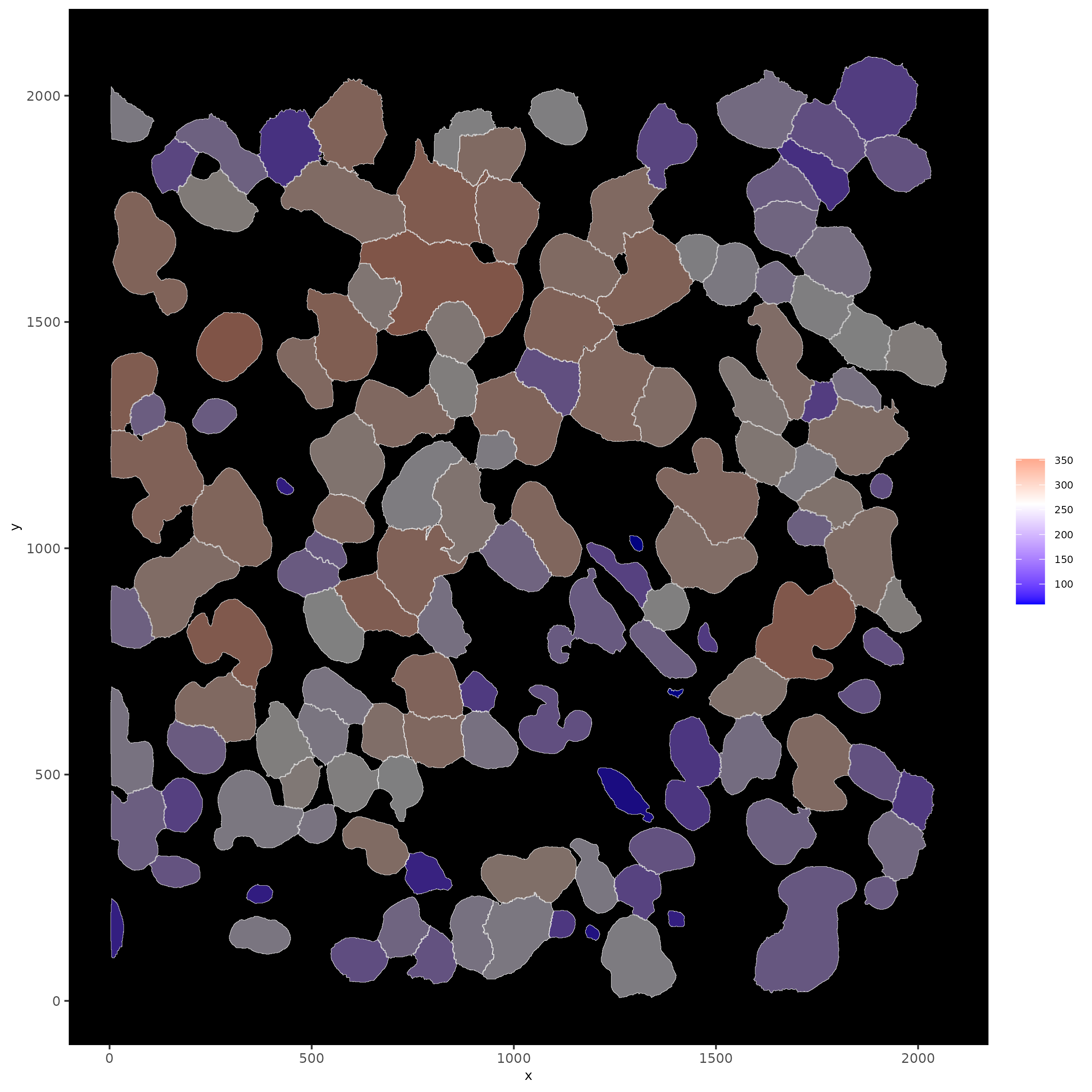```# show cells and color them according to total cluster information
spatInSituPlotPoints(subloc,
show_polygon = TRUE,
polygon_feat_type = 'cell',
polygon_color = 'white',
polygon_line_size = 0.1,
polygon_fill = 'leiden_clus',
polygon_fill_as_factor = T,
polygon_fill_code = colorcode)
```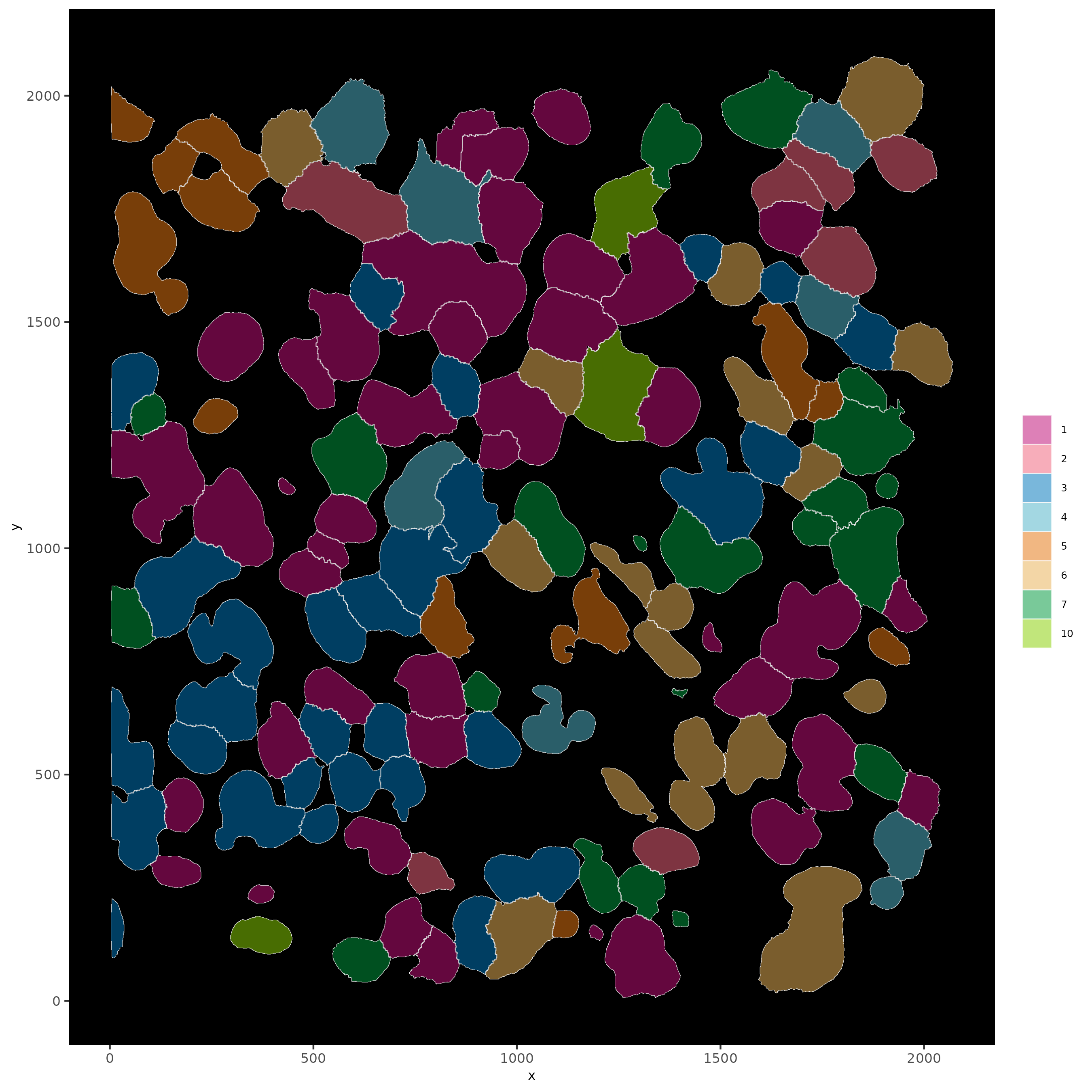## Part 12: Find Interaction Changed Features#

```# find interaction changed Features
# In this case, features are genes whose expression difference is associated with a neighboring cell type
future::plan('multisession', workers = 4) # sometimes unstable, restart R session

test = findInteractionChangedFeats(gobject = subc_test,
cluster_column = 'leiden_clus')

test\$ICFscores[type_int == 'hetero']

spatInSituPlotPoints(subc_test,
feats = list('rna' = c("CTSD", "BMP1")),
point_size = 0.6,
show_polygon = TRUE,
polygon_feat_type = 'cell',
polygon_color = 'black',
polygon_line_size = 0.1,
polygon_fill = 'leiden_clus',
polygon_fill_as_factor = T,
polygon_fill_code = colorcode,
feats_color_code = featcolor)
```